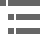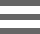## Numerical Aperture and Image ResolutionThe image formed by a perfect, aberration-free objective lens at the intermediate image plane of a microscope is a diffraction pattern produced by spherical waves exiting the rear aperture and converging on the focal point. This tutorial explores the effects of objective numerical aperture on the resolution of the central bright disks present in the diffraction pattern, commonly known as Airy disks.

The Airy pattern formed at the microscope intermediate image plane is a three-dimensional diffraction image, which is symmetrically periodic both along the optical axis of the microscope, and radially across the image plane. This diffraction pattern can be sectioned in the focal plane to produce a two-dimensional diffraction pattern having a bright circular disk surrounded by an alternating series of bright and dark higher-order diffraction rings whose intensity decreases as they become further removed from the central disk. Usually only two or three of the circular luminous rings are visible in the microscope (this number is dependent upon the objective numerical aperture), because the higher orders are absorbed by stray light and are not visible.

To operate the tutorial, used the Numerical Aperture slider to change the objective numerical aperture and the resolution of the Airy patterns in the microscope viewport. The left-hand position of the slider shows the pattern at the lowest objective numerical aperture (0.20), and the right-hand position illustrates the highest degree of resolution (numerical aperture = 1.30) obtainable with this tutorial. Positioned to the right of the microscope port is a three-dimensional representation of an Airy pattern viewed from an angle oblique to the microscope optical axis and the intermediate image plane (commonly referred to as a calculated point spread function). As the slider is moved from left to right, the objective numerical aperture increases and the complex Airy pattern in the view port shrinks, demonstrating a progressively increased resolution of image detail. Simultaneously, the central peak and higher-order diffraction rings in the three-dimensional Airy pattern drawing adjacent to the viewport grow smaller in diameter.

The resolving power of an objective determines the size of the Airy diffraction pattern formed, and the radius of the central disk is determined by the combined numerical apertures of the objective and condenser. When the condenser and objective have equivalent numerical apertures, the Airy pattern radius from the central peak to the first minimum is given by the equation:

where r(Airy) is the Airy radius, λ is the wavelength of illuminating light, and NA(Obj) is the objective (and condenser) numerical aperture. The numerical aperture is dependent upon the angle of the inverted cone of illumination entering the objective aperture, as well as the refractive index of the imaging medium:

where θ is the objective angular aperture and n is the refractive index of the medium (air, water, or oil) between the objective and the specimen. The image resolution, (D) is defined by the equation:

which is clearly influenced by the objective numerical aperture. Note that lower values of indicate higher resolution. In the tutorial, the Numerical Aperture slider is utilized to control how image structure evolves as the objective numerical aperture is increased. At the lowest numerical aperture value (0.20), image details visible in the microscope viewport are poorly defined and surrounded by diffraction fringes that are diffuse, but not resolved. As the slider is moved to higher numerical aperture values (0.50-0.80), the structural outline of the image becomes sharper and higher-order diffraction rings begin to emerge. At the highest numerical apertures (1.00-1.30), diffraction disks become individually resolved as discrete luminous points surrounded by alternating series of bright and dark higher-order diffraction rings of decreasing intensity.

### Contributing Authors

Michael W. Davidson - National High Magnetic Field Laboratory, 1800 East Paul Dirac Dr., The Florida State University, Tallahassee, Florida, 32310.

# Microscopy U - The source for microscopy education•••